# Five-Year Wilkinson Microwave Anisotropy Probe (WMAP) Observations: Cosmological Interpretation

## E. Komatsu, et al., 2009, ApJS, 180, 330-376

### reprint (1.7 MB) / preprint (1.2 MB) / bundled figures (345 KB) / individual figures / ADS / astro-ph

 WMAP Five-year Paper Figures, E. Komatsu, et al. Individual figures are provided for use in talks. Proper display of PNG transparency in PowerPoint requires saving files to your computer before Inserting them. Please acknowledge the WMAP Science Team when using these images. Image Credit: NASA / WMAP Science Team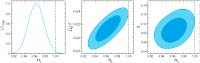Fig. 01Constraint on the primordial tilt, ns (ξ 3.1.2). No running index or gravitational waves are included in the analysis. (Left) One-dimensional marginalized constraint on ns from the WMAP-only analysis. (Middle) Two-dimensional joint marginalized constraint (68% and 95% CL), showing a strong degeneracy between ns and Ωbh2. (Right) A mild degeneracy with τ . None of these degeneracies improve signifcantly by including BAO or SN data, as these data sets are not sensitive to Ωbh2 or τ however, the situation changes when the gravitational wave contribution is included (see Fig. 3). PNG (17 kb - 642x2048 pixels) PNG (39 kb - 1284x4096 pixels) EPS (47 kb)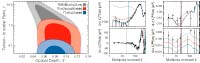Fig. 02How the WMAP temperature and polarization data constrain the tensor-to-scalar ratio, r. (Left) The contours show 68% and 95% CL. The gray region is derived from the low-l polarization data (TE/EE/BB at l ≤ 23) only, the red region from the low-l polarization plus the high-l TE data at l ≤ 450, and the blue region from the low-l polarization, the high-l TE, and the low-l temperature data at l ≤ 32. (Right) The gray curves show (r, τ ) = (10, 0.050), the red curves (r, τ ) = (1.2, 0.075), and the blue curves (r, τ ) = (0.20, 0.080), which are combinations of r and τ that give the upper edge of the 68% CL contours shown on the left panel. The vertical lines indicate the maximum multipoles below which the data are used for each color. The data points with 68% CL errors are the WMAP 5-year measurements (Nolta et al. 2008). (Note that the BB power spectrum at l ~ 130 is consistent with zero within 95% CL.) PNG (29 kb - 642x2048 pixels) PNG (67 kb - 1284x4096 pixels) EPS (127 kb)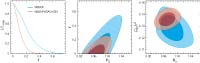Fig. 03Constraint on the tensor-to-scalar ratio, r, at k = 0.002 Mpc-1 (ξ 3.2.4). No running index is assumed. See Fig. 4 for r with the running index. In all panels we show the WMAP-only results in blue and WMAP+BAO+SN in red. (Left) One-dimensional marginalized distribution of r, showing the WMAP-only limit, r < 0.43 (95% CL), and WMAP+BAO+SN, r < 0.20 (95% CL). (Middle) Joint two-dimensional marginalized distribution (68% and 95% CL), showing a strong degeneracy between ns and r. (Right) Degeneracy between ns and Ωmh2. The BAO and SN data help to break this degeneracy which, in turn, reduces the degeneracy between r and ns, resulting in a factor of 2.2 better limit on r. PNG (19 kb - 642x2048 pixels) PNG (43 kb - 1284x4096 pixels) EPS (53 kb)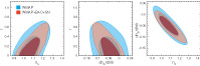Fig. 04Constraint on the tensor-to-scalar ratio, r, the tilt, ns, and the running index, dns/d ln k, when all of them are allowed to vary (ξ 3.2.4). In all panels we show the WMAP-only results in blue and WMAP+BAO+SN in red. (Left) Joint two-dimensional marginalized distribution of ns, and r at k = 0.002 Mpc-1 (68% and 95% CL). (Middle) ns and dns/d ln k. (Right) dns/d ln k and r. We find no evidence for the running index. While the inclusion of the running index weakens our constraint on ns, and r, the data do not support any need for treating the running index as a free parameter. PNG (23 kb - 679x2048 pixels) PNG (46 kb - 1357x4096 pixels) EPS (55 kb)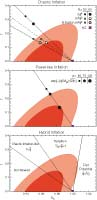Fig. 05Constraint on three representative inflation models whose potential is positively curved, V″ > 0 (ξ 3.3). The contours show the 68% and 95% CL derived from WMAP+BAO+SN. (Top) The monomial, chaotic-type potential, V(φ) ∝ φ∝ (Linde 1983), with α = 4 (solid) and α = 2 (dashed) for single-field models, and α = 2 for multi-axion field models with β = 1/2 (Easther & McAllister 2006) (dotted). The symbols show the predictions from each of these models with the number of e-folds of inflation equal to 50 and 60. The λφ4 potential is excluded convincingly. (Middle) The exponential potential, V (Φ) ∝ exp[-(Φ/Mpl)√2/p], which leads to a power-law inflation, a(t) ∝ tp (Abbott & Wise 1984; Lucchin & Matarrese 1985). All models but p ~ 120 are outside of the 68% region. For multi-field models these limits can be translated into the number of fields as P → npi, where pi is the p-parameter of each field (Liddle et al. 1998), the data favour n ~ 120/pi fields, where pi is the p-parameter of each field. (Bottom) The hybrid-type potential, V(φ) =V0 + (1/2)m2φ2 = V0(1 + φ2), where φ ≡ mφ/(2V0)1/2 (Linde 1994). The models with φ < 2/3 drive inflation by the vacuum energy term, V0, and are disfavoured at more than 95% CL, while those with φ > 1 drive inflation by the quadratic term, and are similar to the chaotic type (the left panel with α = 2). The transition regime, 2/3 < φ < 1 are outside of the 68% region, but still within the 95% region. PNG (29 kb - 1024x2121 pixels) PNG (71 kb - 2048x4242 pixels) EPS (76 kb)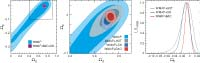Fig. 06Joint two-dimensional marginalized constraint on the vacuum energy density, ΩΛ, and the spatial curvature parameter, Ωk (ξ 3.4.3). The contours show the 68% and 95% CL. (Left) The WMAP-only constraint (light blue) compared with WMAP+BAO+SN (purple). Note that we have a prior on ΩΛ, ΩΛ > 0. This figure shows how powerful the extra distance information is for constraining Ωk. (Middle) A blow-up of the region within the dashed lines in the left panel, showing WMAP-only (light blue), WMAP+HST (gray), WMAP+SN (dark blue), and WMAP+BAO (red). The BAO provides the most stringent constraint on Ωk. (Right) One-dimensional marginalized constraint on Ωk from WMAP+HST, WMAP+SN, and WMAP+BAO. We find the best limit, -0.0181 < Ωk < 0.0071 (95% CL), from WMAP+BAO+SN, which is essentially the same as WMAP+BAO. See Fig. 12 for the constraints on Ωk when dark energy is dynamical, i.e., w ≠-1, with time-independent w. PNG (25 kb - 642x2048 pixels) PNG (58 kb - 1284x4096 pixels) EPS (65 kb)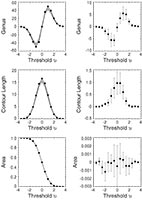Fig. 07Minkowski functionals from the WMAP 5-year data, measured from the template-cleaned V+W map at Nside = 128 (28' pixels) outside of the KQ75 mask. From the top to bottom panels, we show the Euler characteristics (also known as the genus), the contour length, and the cumulative surface area, as a function of the threshold (the number of σ's of hot and cold spots), ν≡ ΔT/σ0. (Left) The data (symbols) are fully consistent with the mean and dispersion of Gaussian realizations that include CMB and noise. The gray bands show the 68% intervals of Gaussian realizations. (Right) The residuals between the WMAP data and the mean of the Gaussian realizations. PNG (22 kb - 1024x1442 pixels) PNG (53 kb - 2048x2885 pixels) EPS (98 kb)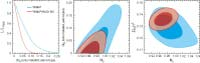Fig. 08Constraint on the axion entropy perturbation fraction, α0 (ξ 3.6.3). In all panels we show the WMAP-only results in blue and WMAP+BAO+SN in red. (Left) One-dimensional marginalized constraint on α0, showing WMAP-only and WMAP+BAO+SN. (Middle) Joint two-dimensional marginalized constraint (68% and 95% CL), showing the degeneracy between α0 and ns for WMAP-only and WMAP+BAO+SN. (Right) Degeneracy between ns and Ωmh2. The BAO and SN data help to break this degeneracy which, in turn, reduces degeneracy between α0 and ns, resulting in a factor of 2.4 better limit on α0. PNG (21 kb - 646x2048 pixels) PNG (50 kb - 1292x4096 pixels) EPS (92 kb)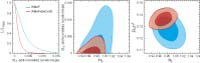Fig. 09Constraint on the curvaton entropy perturbation fraction, α-1 (ξ 3.6.4). In all panels we show the WMAP-only results in blue and WMAP+BAO+SN in red. (Left) One-dimensional marginalized constraint on α-1, showing WMAP-only and WMAP+BAO+SN. (Middle) Joint two-dimensional marginalized constraint (68% and 95% CL), showing the degeneracy between α-1 and ns for WMAP-only and WMAP+BAO+SN. (Right) Degeneracy between ns and Ωmh2. The BAO and SN data help to break this degeneracy which, in turn, reduces degeneracy between α-1 and ns, resulting in a factor of 3 better limit on α-1. These properties are similar to those of the axion dark matter presented in Fig. 8. PNG (21 kb - 646x2048 pixels) PNG (49 kb - 1292x4096 pixels) EPS (74 kb)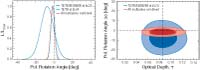Fig. 10Constraint on the polarization rotation angle, Δα, due to a parity-violating interaction that rotates the polarization angle of CMB (ξ 4.3). We have used the polarization spectra (TE/TB/EE/BB/EB at l ≤ 23, and TE/TB at l ≥ 24), and did not use the TT power spectrum. (Left) One-dimensional marginalized constraint on Δα in units of degrees. The dark blue, light blue, and red curves show the limits from the low-l (2 ≤ l ≤ 23), high-l (24 ≤ l ≤ 450), and combined (2 ≤ l ≤ 450) analysis of the polarization data, respectively. (Right) Joint two-dimensional marginalized constraint on τ and Δα (68% and 95% CL). The bigger contours are from the low-l analysis, while the smaller ones are from the combined analysis. The vertical dotted line shows the best-fitting optical depth in the absence of parity violation (τ = 0.086), whereas the horizontal dotted line shows Δα = 0 to guide eyes. PNG (18 kb - 642x1845 pixels) PNG (42 kb - 1284x3690 pixels) EPS (76 kb)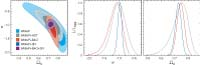Fig. 11Constraint on the time-independent (constant) dark energy equation of state, w, and the present-day dark energy density, ΩΛ, assuming a flat universe, Ωk = 0 (ξ 5.2). Note that we have imposed a prior on w, w > -2.5. (Left) Joint two-dimensional marginalized distribution of w and Ωk. The contours show the 68% and 95% CL. The WMAP-only constraint (light blue) is compared with WMAP+HST (gray), WMAP+BAO (red), WMAP+SN (dark blue), and WMAP+BAO+SN (purple). This figure shows how powerful a combination of the WMAP data and the current SN data is for constraining w. (Middle) One-dimensional marginalized constraint on w for a flat universe from WMAP+HST (gray), WMAP+BAO (red), and WMAP+SN (dark blue). The WMAP+BAO+SN result (not shown) is essentially the same as WMAP+SN. (Right) One-dimensional marginalized constraints on w for a flat universe from WMAP+HST (gray), WMAP+BAO (red), and WMAP+SN (dark blue). PNG (25 kb - 670x2048 pixels) PNG (59 kb - 1341x4096 pixels) EPS (72 kb)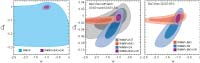Fig. 12Joint two-dimensional marginalized constraint on the time-independent (constant) dark energy equation of state, w, and the curvature parameter, Ωk (ξ 5.3). Note that we have imposed a prior on w, w > -2.5. The contours show the 68% and 95% CL. (Left) The WMAP-only constraint (light blue; 95% CL) compared with WMAP+BAO+SN (purple; 68% and 95% CL). This figure shows how powerful the extra distance information from BAO and SN is for constraining Ωk and w simultaneously. (Middle) A blow-up of the left panel, showing WMAP+HST (gray), WMAP+BAO (red), WMAP+SN (dark blue), and WMAP+BAO+SN (purple). This figure shows that we need both BAO and SN to constrain Ωk and w simultaneously: WMAP+BAO fixes Ωk, and WMAP+SN fixes w. (Right) The same as the middle panel, but with the BAO prior re-weighted by a weaker BAO prior from the SDSS LRG sample (Eisenstein et al. 2005). The BAO data used in the other panels combine the SDSS main and LRG, as well as the 2dFGRS data (Percival et al. 2007). The constraints from these are similar, and thus our results are not sensitive to the exact form of the BAO data sets. PNG (23 kb - 642x2048 pixels) PNG (50 kb - 1284x4096 pixels) EPS (117 kb)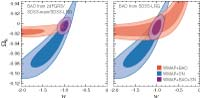Fig. 13Joint two-dimensional marginalized constraint on the time-independent (constant) dark energy equation of state, w, and the curvature parameter, Ωk, derived solely from the WMAP distance priors (lA, R, z*) (see ξ 5.4.1), combined with either BAO (red) or SN (dark blue) or both (purple). The contours show the ΔX2 total = 2.30 (68.3% CL) and ΔX2 total = 6.17 (95.4% CL). The left (BAO data from Percival et al. (2007)) and right (BAO data from Eisenstein et al. (2005)) panels should be compared with the middle and right panels of Fig. 12, respectively, which have been derived from the full WMAP data combined with the same BAO and SN data. While the WMAP distance priors capture most of the information in this parameter space, the constraint is somewhat weaker than that from the full analysis. PNG (15 kb - 642x1317 pixels) PNG (34 kb - 1284x2633 pixels) EPS (77 kb)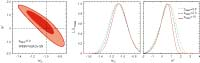Fig. 14Constraint on models of time-dependent dark energy equation of state, w(z) (Eq. ), derived from the WMAP distance priors (lA, R, and z*) combined with the BAO and SN distance data (ξ 5.4.2). There are three parameters: w0 is the value of w at the present epoch, w0 ≡ w(z = 0), w' is the first derivative of w with respect to z at z = 0, w' ≡ dw/dz|z=0, and ztrans is the transition redshift above which w(z) approaches to -1. Here, we assume flatness of the universe, Ωk = 0. (Left) Joint two-dimensional marginalized distribution of w0 and w' for ztrans = 10. The constraints are similar for the other values of ztrans. The contours show the ΔX2 total = 2.30 (68.3% CL) and ΔX2 total = 6.17 (95.4% CL). (Middle) One-dimensional marginalized distribution of w0 for ztrans = 0.5 (dotted), 2 (dashed), and 10 (solid). (Middle) One-dimensional marginalized distribution of w0 for ztrans = 0.5 (dotted), 2 (dashed), and 10 (solid). The constraints are similar for all ztrans. We do not find evidence for the evolution of dark energy. PNG (21 kb - 642x2048 pixels) PNG (49 kb - 1284x4096 pixels) EPS (47 kb)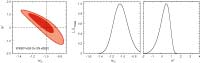Fig. 15Constraint on the linear evolution model of dark energy equation of state, w(z) = w0 + w' z/(1 + z), derived from the WMAP distance priors (lA, R, and z*) combined with the BAO and SN distance data as well as the Big Bang Nucleosynthesis (BBN) prior (Eq. ). Here, we assume flatness of the universe, Ωk = 0. (Left) Joint two-dimensional marginalized distribution of w0 and w'. The contours show the ΔX2 total = 2.30 (68.3% CL) and ΔX2 total = 6.17 (95.4% CL). (Middle) One-dimensional marginalized distribution of w0. (Middle) One-dimensional marginalized distribution of w'. We do not find evidence for the evolution of dark energy. Note that Linder (2003) defines w' as the derivative of w at z = 1, whereas we define it as the derivative at z = 0. They are related by w'linder = 0.5w'WMAP. PNG (15 kb - 642x2048 pixels) PNG (36 kb - 1284x4096 pixels) EPS (36 kb)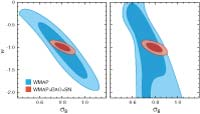Fig. 16Predictions for the present-day amplitude of matter fluctuations, σ8, as a function of the (constant) dark energy equation of state parameter, w, derived from the full WMAP data (blue) as well as from WMAP+BAO+SN (red). The contours show the 68% and 95% CL. (Left) Flat universe, Ωk = 0. (Right) Curved universe, Ωk≠0. PNG (9 kb - 585x1024 pixels) PNG (21 kb - 1170x2048 pixels) EPS (22 kb)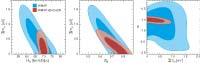Fig. 17Constraint on the total mass of neutrinos, Σmν (ξ 6.1.3). In all panels we show the WMAP-only results in blue and WMAP+BAO+SN in red. (Left) Joint two-dimensional marginalized distribution of H0 and Σmν (68% and 95% CL). The additional distance information from BAO helps reduce the degeneracy between H0 and Σmν. (Middle) The WMAP data, combined with the distances from BAO and SN, predict the present-day amplitude of matter fluctuations, σ8, as a function of Σmν . An independent determination of σ8 will help determine Σmν tremendously. (Right) Joint two-dimensional marginalized distribution of w and Σmν. No significant degeneracy is observed. Note that we have a prior on w, w > -2.5, and thus the WMAP-only lower limit on w in this panel cannot be trusted. PNG (20 kb - 642x2048 pixels) PNG (47 kb - 1284x4096 pixels) EPS (52 kb)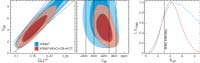Fig. 18Constraint on the effective number of neutrino species, Neff (ξ 6.2.3). Note that we have imposed a prior on Neff, 0 < Neff < 10. In all panels we show the WMAP-only results in blue and WMAP+BAO+SN in red. (Left) Joint two-dimensional marginalized distribution (68% and 95% CL), showing a strong degeneracy between Ωm h2 and Neff. This degeneracy line is given by the equality redshift, 1+zeq = Ωm/Ωr =(4.050 x 104 Ωmh2/(1+0.2271Neff). The thick solid lines show the 68% and 95% limits calculated from the WMAP-only limit on zeq: zeq = 3141+154-157 (68%CL). The 95% CL contours do not follow the lines below Neff ~ 1.5 but close there, which shows strong evidence for the cosmic neutrino background from its effects on the CMB power spectrum via the neutrino anisotropic stress. The BAO and SN provide an independent constraint on Ωmh2, which helps reduce the degeneracy between Neff and Ωmh2. (Middle) When we transform the horizontal axis of the left panel to zeq, we observe no degeneracy. (Right) One-dimensional marginalized distribution of Neff from WMAP-only and WMAP+BAO+SN+HST. Note that a gradual decline of the likelihood toward N eff ≥∼ 6 for the WMAP-only constraint should not be trusted, as it is affected by the hard prior, Neff < 10. The WMAP+BAO+SN+HST constraint is robust. This figure shows that the lower limit on Neff is coming solely from the WMAP data. The 68% interval from WMAP+BAO+SN+HST, Neff = 4.4 ± 1.5, is consistent with the standard value, 3.04 (vertical line). PNG (24 kb - 650x2048 pixels) PNG (58 kb - 1300x4096 pixels) EPS (53 kb)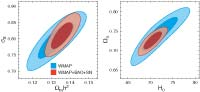Fig. 19Four representative cosmological parameters that have improved significantly by adding the BAO and SN data. (See also Table 1.) The contours show the 68% and 95% CL. The WMAP-only constraint is shown in blue, while WMAP+BAO+SN in red. (Left) The distance information from BAO and SN provides a better determination of Ωmh2, which results in 40% better determination of σ8. (Right) The BAO data, being the absolute distance indicator, provides a better determination of H0, which results in a factor of 2 better determination of ΩΛ, Ωb, and Ωc. PNG (13 kb - 642x1394 pixels) PNG (30 kb - 1284x2788 pixels) EPS (41 kb)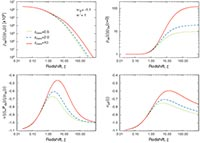Fig C1Evolution of dark energy for w0 = -1.1 and w' = 1, and various transition redshifts, ztrans = 0.5, 2, and 10, above which w(z) approaches to -1. (Top Left) Evolution of the dark energy to matter density ratio as a function of z. Note that the vertical axis has been multiplied by 106. (Top Right) Evolution of the dark energy density relative to the dark energy density at present. The dark energy density was nearly constant at high redshifts above ztrans; thus, these models can describe the "thawing models," (Caldwell & Linder 2005) in which dark energy was nearly constant at early times, and had become dynamical at lower redshifts. (Bottom Left) Evolution of the equation of state, w(z) = Pde(z)/ρde(z). By construction of the model, w(z) approaches to -1 beyond ztrans. (Bottom Right) Evolution of the effective equation of state, weff(z), which determines the evolution of dark energy density as ρde(z) = ρde(0)(1 + z)3[ 1+weff(z)]. PNG (41 kb - 1288x1804 pixels) PNG (88 kb - 2576x3608 pixels) EPS (100 kb)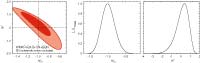Fig D1The same as Fig. 15, but the systematic errors in the Type Ia supernova data are included. PNG (16 kb - 642x2048 pixels) PNG (38 kb - 1284x4096 pixels) EPS (43 kb)
A service of the HEASARC and of the Astrophysics Science Division at NASA/GSFCHEASARC Director: Dr. Alan P. Smale
LAMBDA Director: Dr. Eric R. Switzer
NASA Official: Dr. Eric R. Switzer
Web Curator: Mr. Michael R. Greason